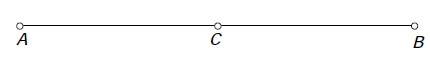Welcome to Geometry
substitute correctly and solve. *
1 point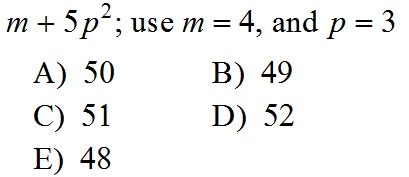What type of angle is this? *
1 point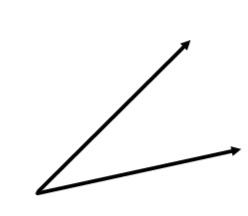Identify what type of angle this measure would be *
1 point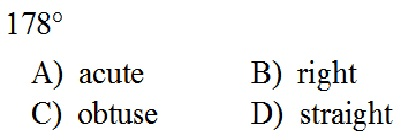What is the name of a 5 sided polygon? *
1 point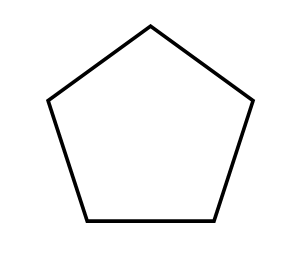Last Name *
Find missing angle on this isosceles triangle *
1 point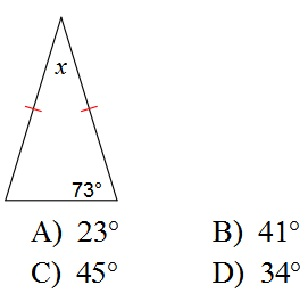Solve for n. *
1 point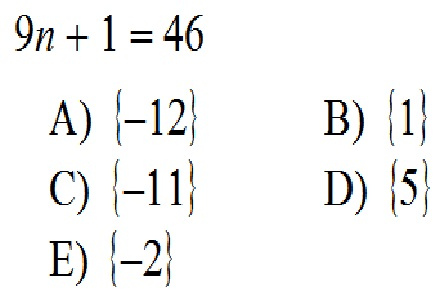solve for a. *
1 point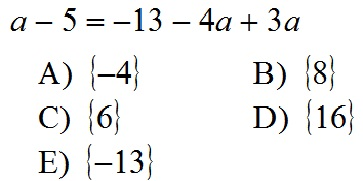What type of angle is this? *
1 point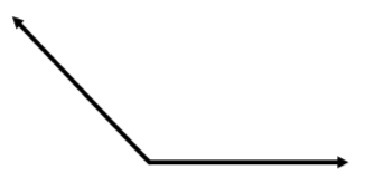Find the missing angle of the triangle *
1 point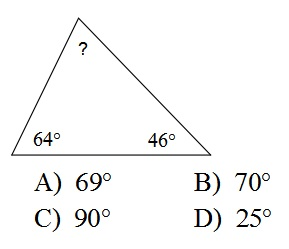How many sides does a octagon have? *
1 point
Every angle in a rectangle is.... *
1 point
Find missing angle in this equilateral triangle *
1 point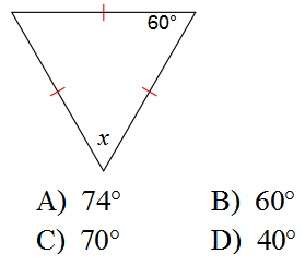Period *
Find missing angle in this Quadrilateral.. Four sided shapes have 360 degrees in them *
1 point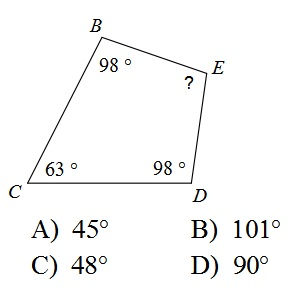Identify what this red line is *
1 point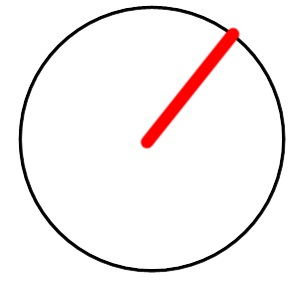Identify this point *
1 point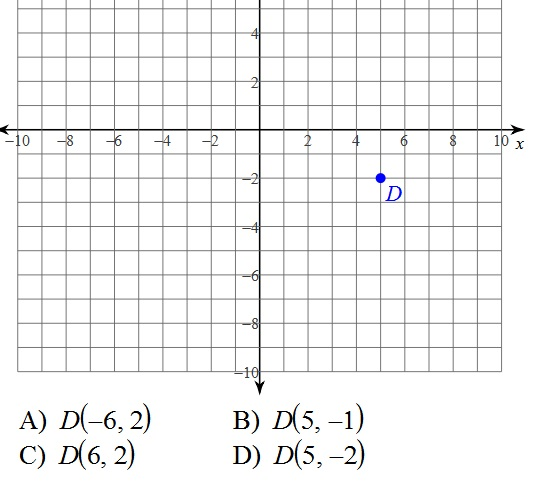Solve for x. *
1 point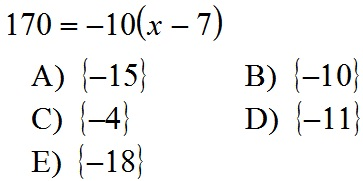Solve for v. *
1 point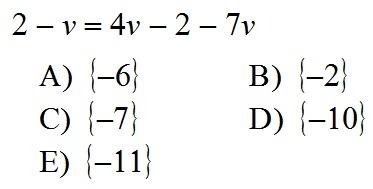Combine like terms *
1 point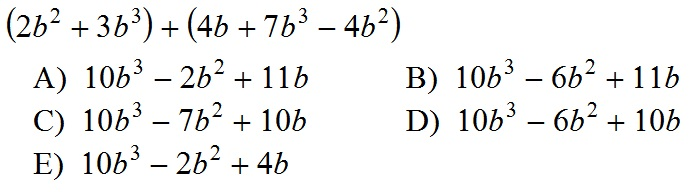Find perimeter *
1 point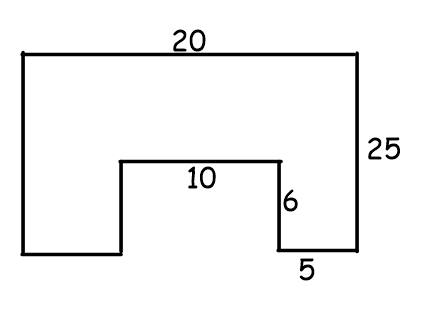C is the midpoint of segment AB. If segment AB is 12 inches. How long is segment CB? *
1 point